« Back to curriculum

# Homeschool Math Curriculum

Choosing a homeschool math curriculum can be a difficult process. Which math curriculum will be best for your family? Which curriculum will be the easiest to teach? What do other homeschoolers have to say?

This page lists the most popular homeschool math curriculum options. Each product includes important information such as the grades covered, the method used, the required parent involvement, associated world views, and more.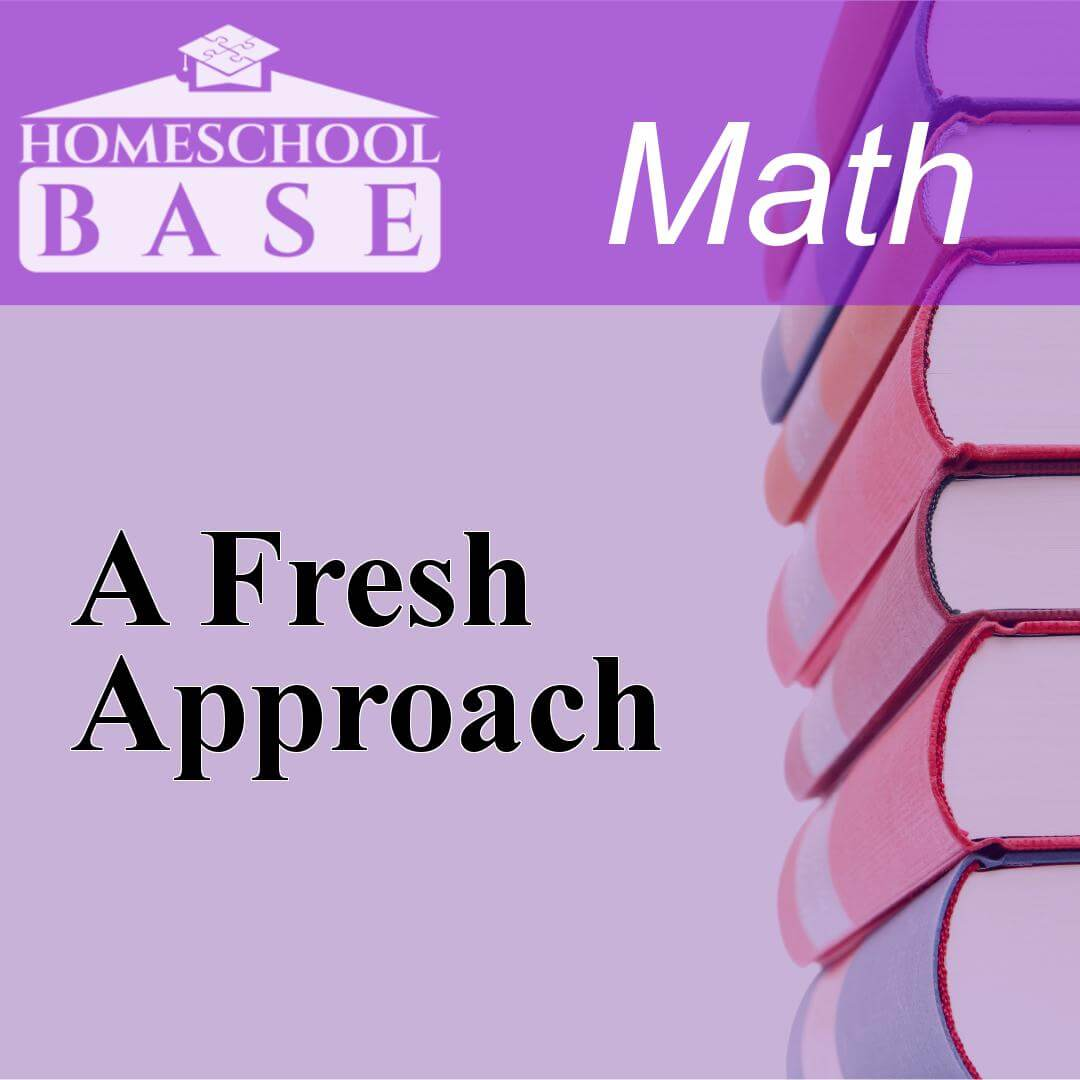## A Fresh Approach

A Fresh Approach is a Math curriculum for grades 8-12. This program teaches by using the sequential method. The average amount of teacher involvement on the parent(s) is: low. This curriculum does not...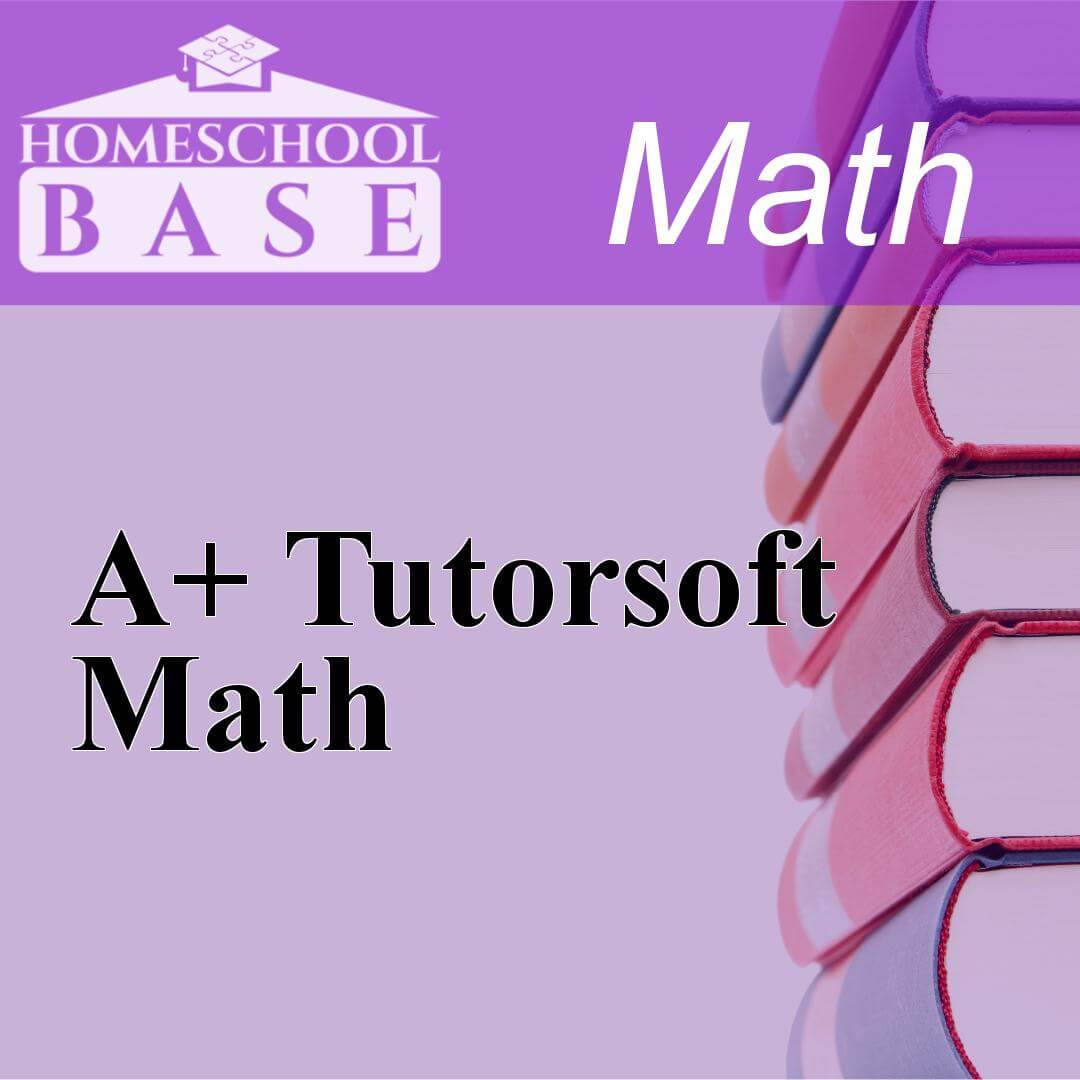## A+ Tutorsoft Math

A+ Tutorsoft Math is a Math curriculum for grades 1-9. This program teaches by using the sequential method. The average amount of teacher involvement on the parent(s) is: low. This curriculum does not...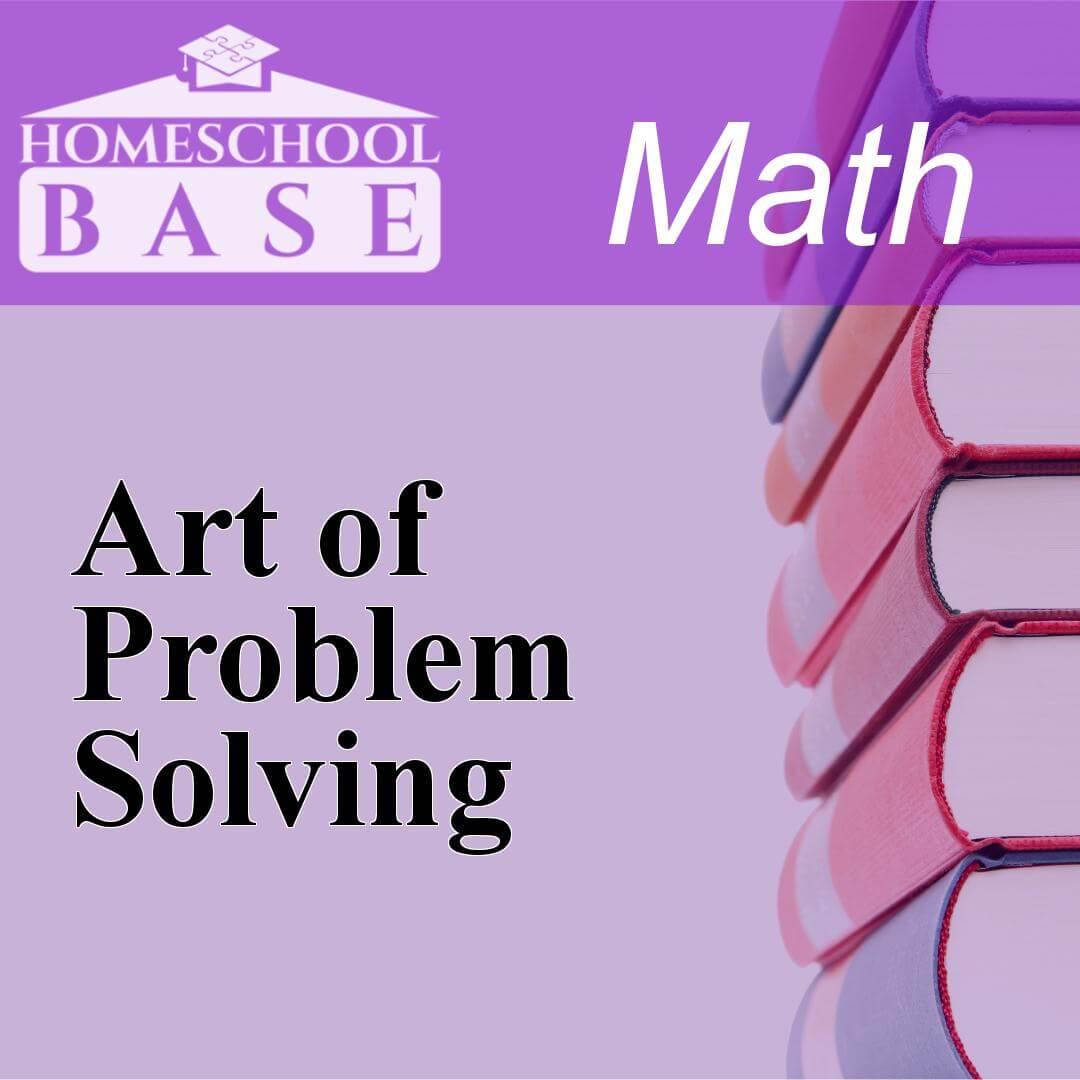## Art of Problem Solving

Art of Problem Solving is a Math curriculum for grades 6-12. This program teaches by using the sequential method. The average amount of teacher involvement on the parent(s) is: low. This curriculum d...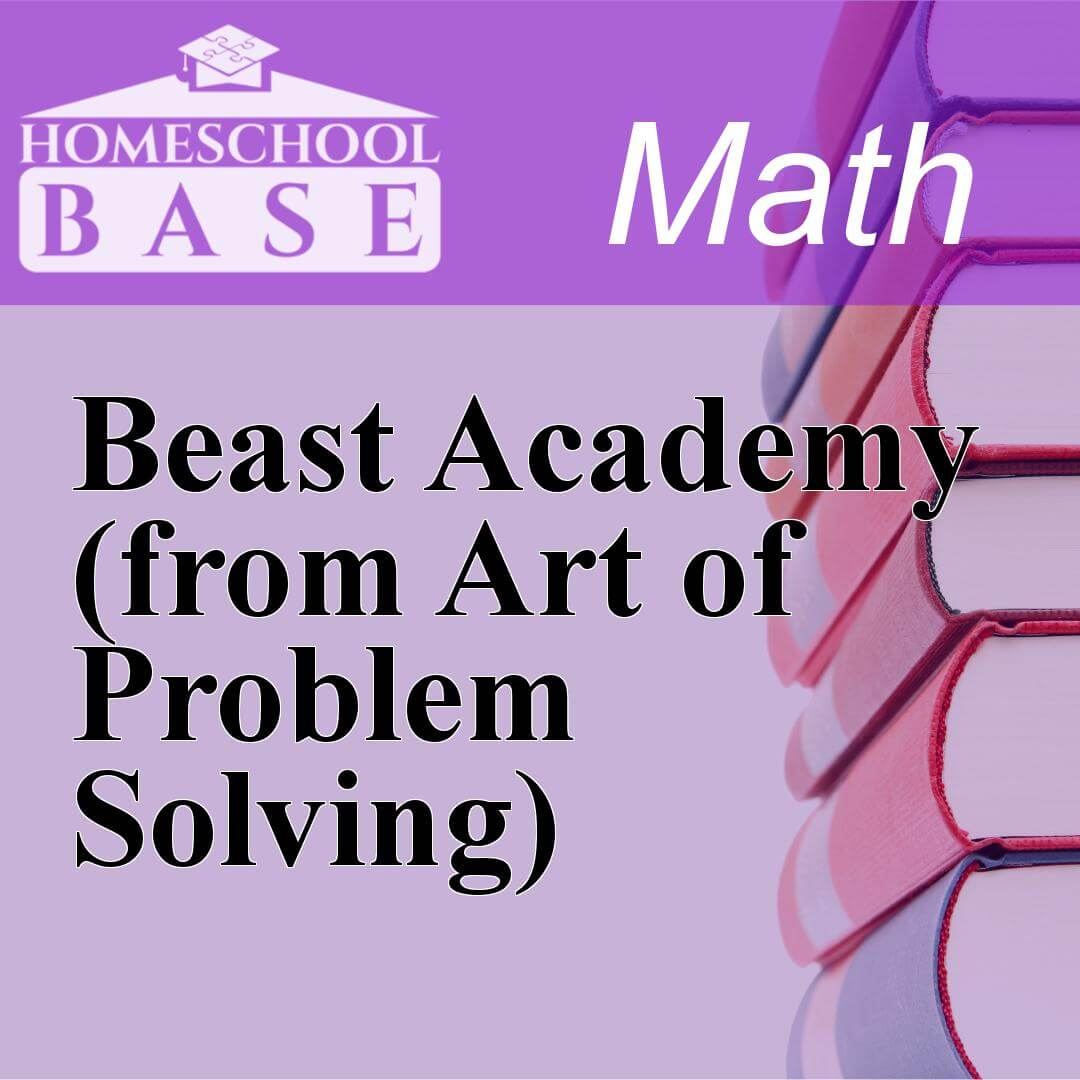## Beast Academy (from Art of Problem Solving)

Beast Academy (from Art of Problem Solving) is a Math curriculum for grades 2-5. This program teaches by using the conceptual-topical method. The average amount of teacher involvement on the parent(s)...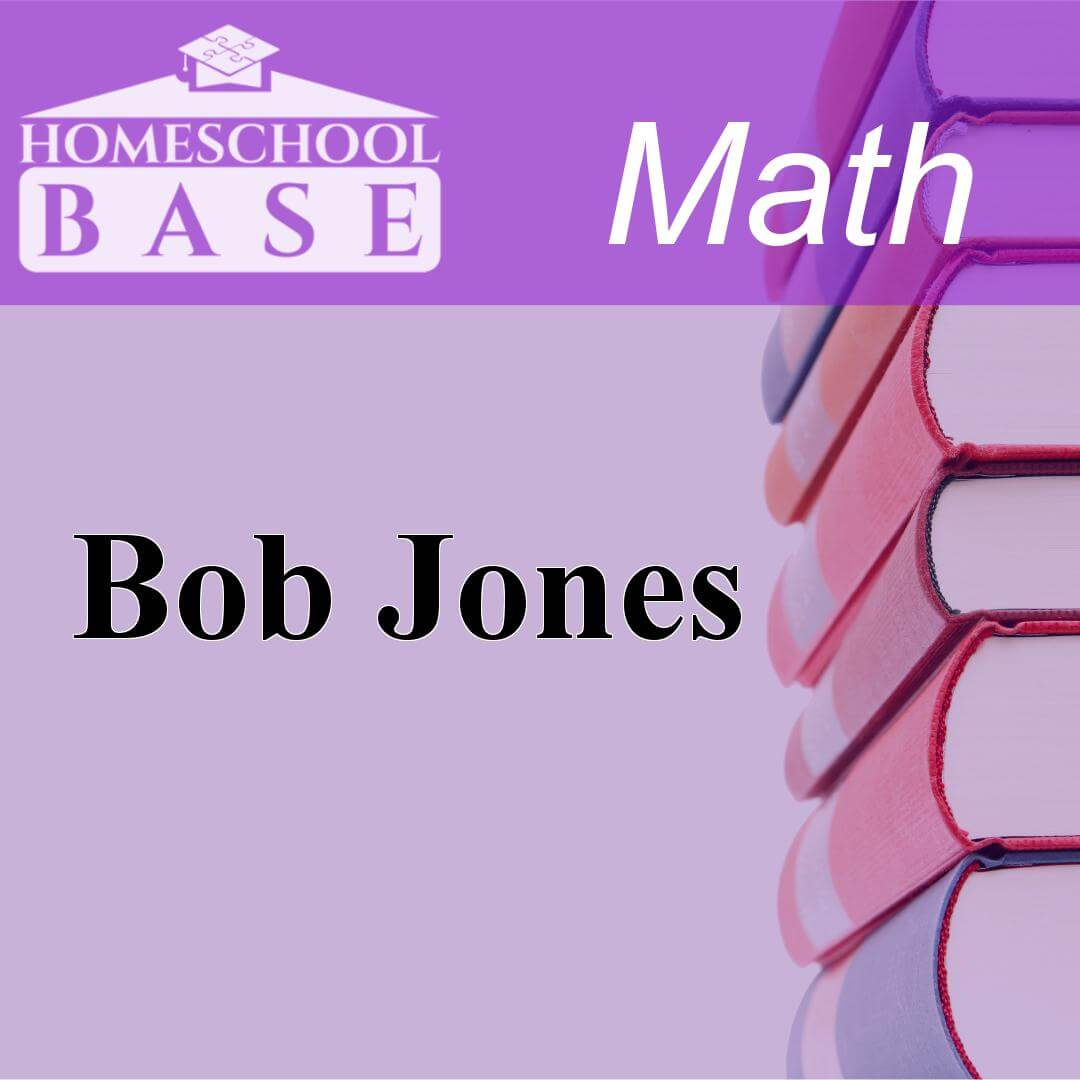## Bob Jones

Bob Jones is a Math curriculum for grades K-12. This program teaches by using the spiral method. The average amount of teacher involvement on the parent(s) is: high. This curriculum does not conform/a...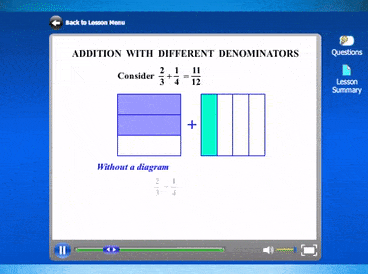## CTC Math Curriculum

CTC Math gives homeschoolers access to all lessons across all grade levels. Levels span from Kindergarten to Trigonometry. CTC Math provides a full mathematics curriculum for K-8. After 8th grade, CTC...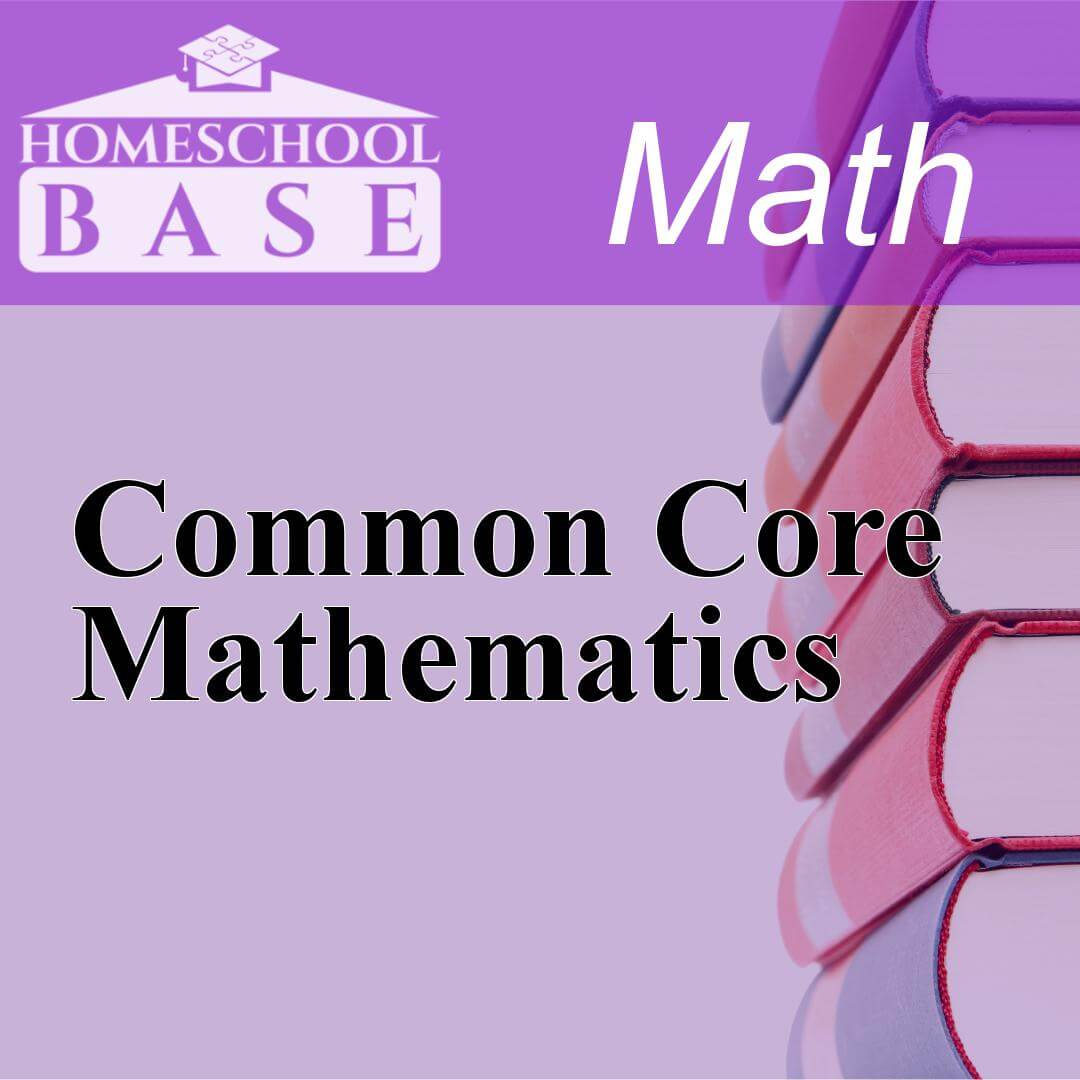## Common Core Mathematics

Common Core Mathematics is a Math curriculum for grades K-6. This program teaches by using the sequential method. The average amount of teacher involvement on the parent(s) is: medium. This curriculum...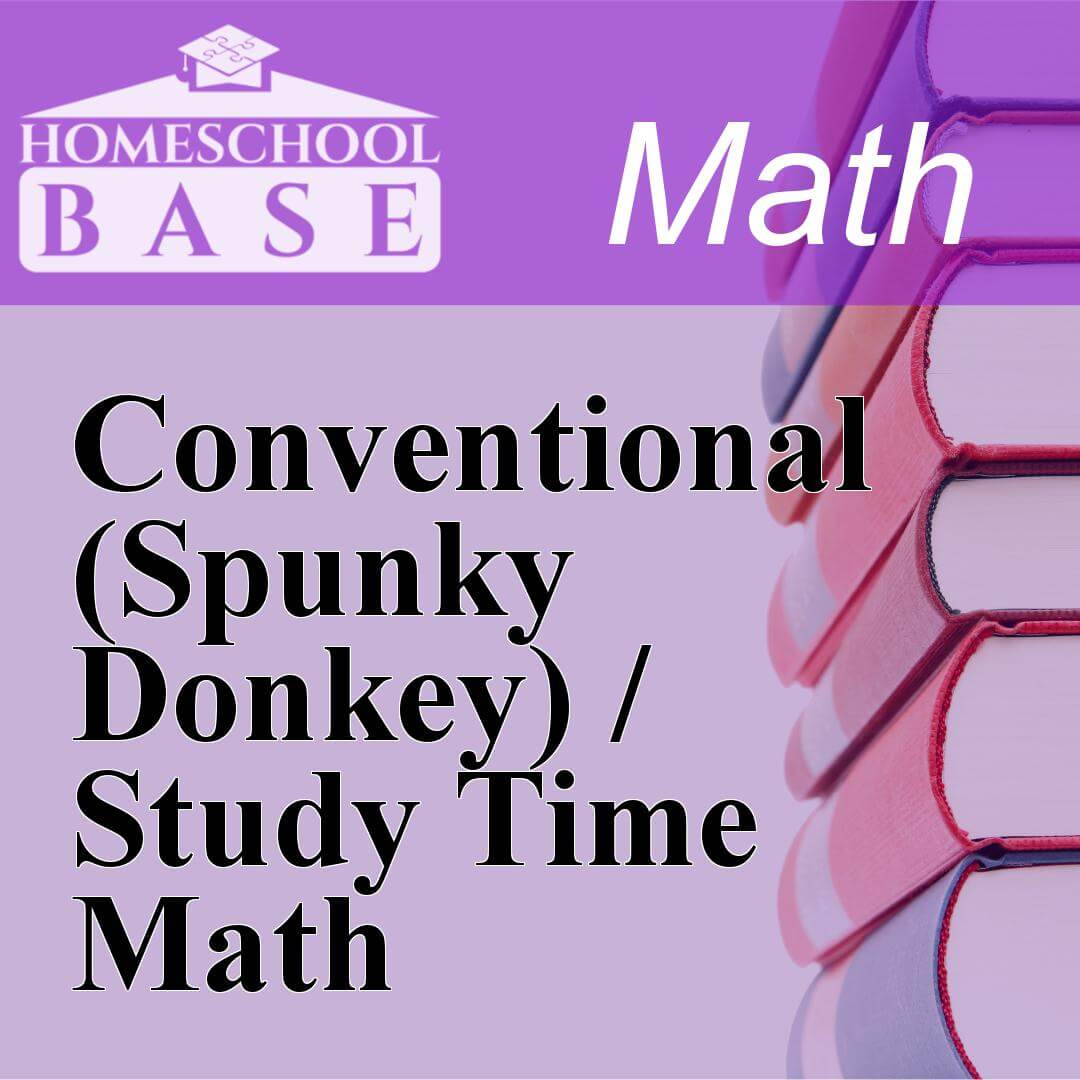## Conventional (Spunky Donkey) / Study Time Math

Conventional (Spunky Donkey) / Study Time Math is a Math curriculum for grades 1-8. This program teaches by using the spiral method. The average amount of teacher involvement on the parent(s) is: medi...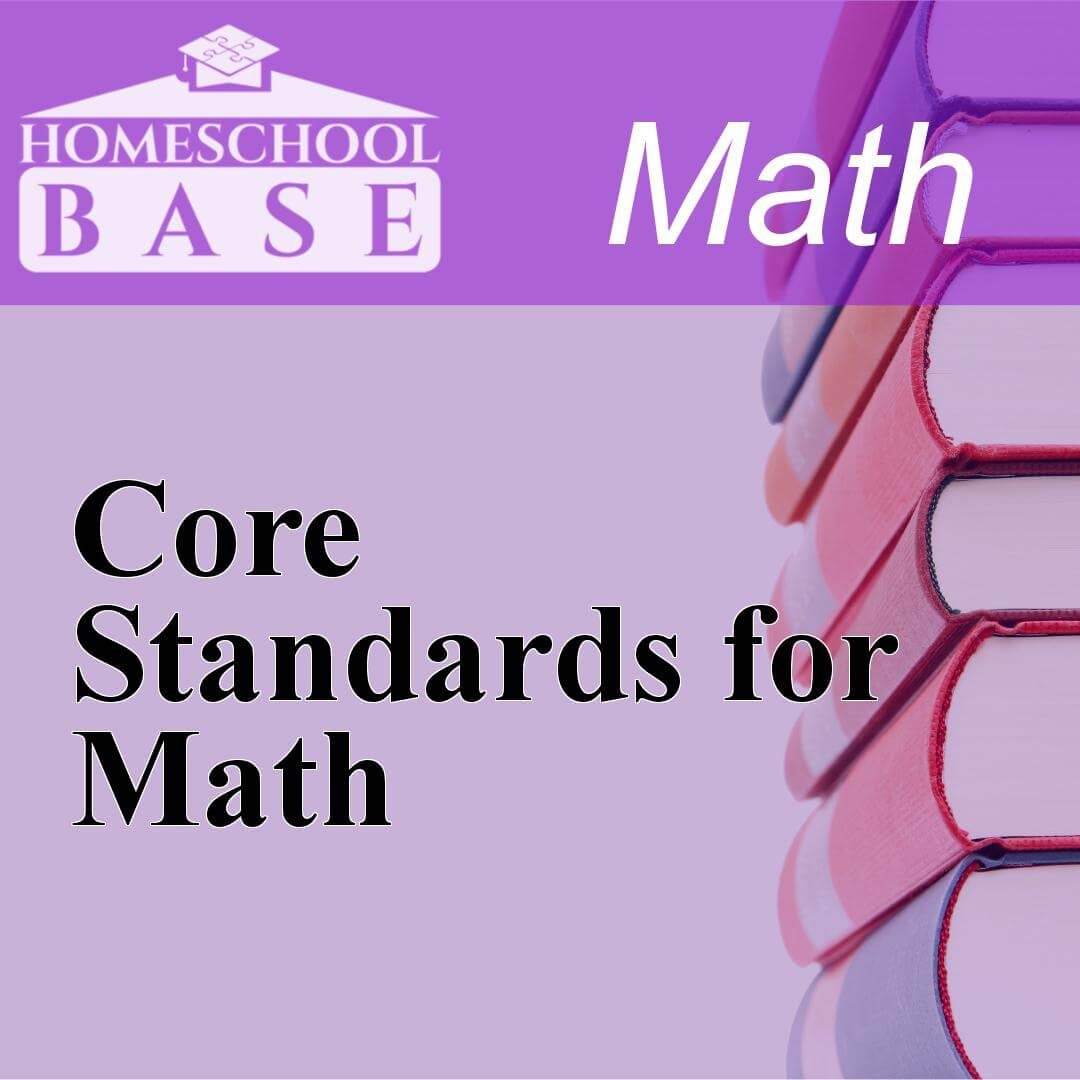## Core Standards for Math

Core Standards for Math is a Math curriculum for grades K-6. This program teaches by using the sequential method. The average amount of teacher involvement on the parent(s) is: medium. This curriculum...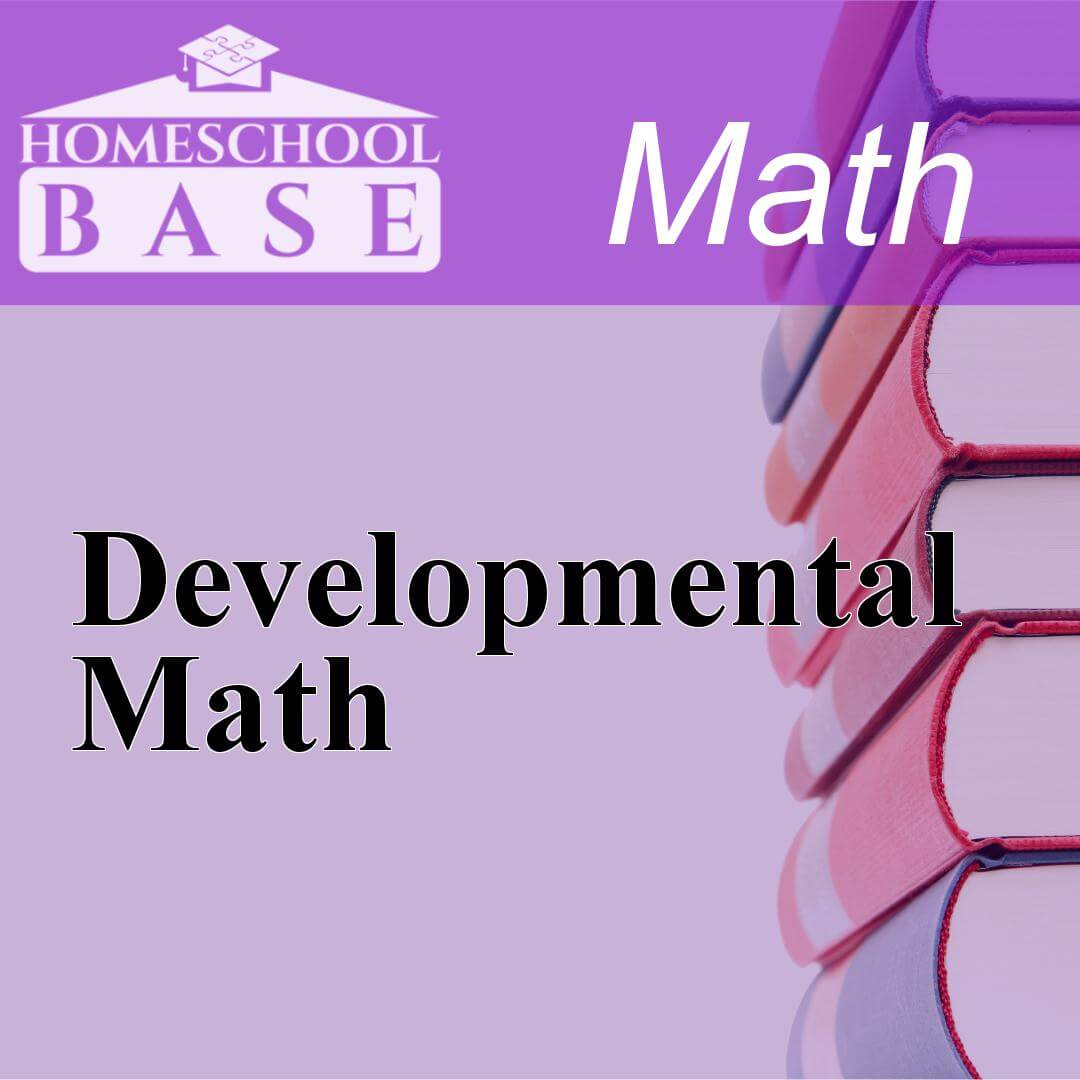## Developmental Math

Developmental Math is a Math curriculum for grades K-8. This program teaches by using the sequential method. The average amount of teacher involvement on the parent(s) is: low. This curriculum does no...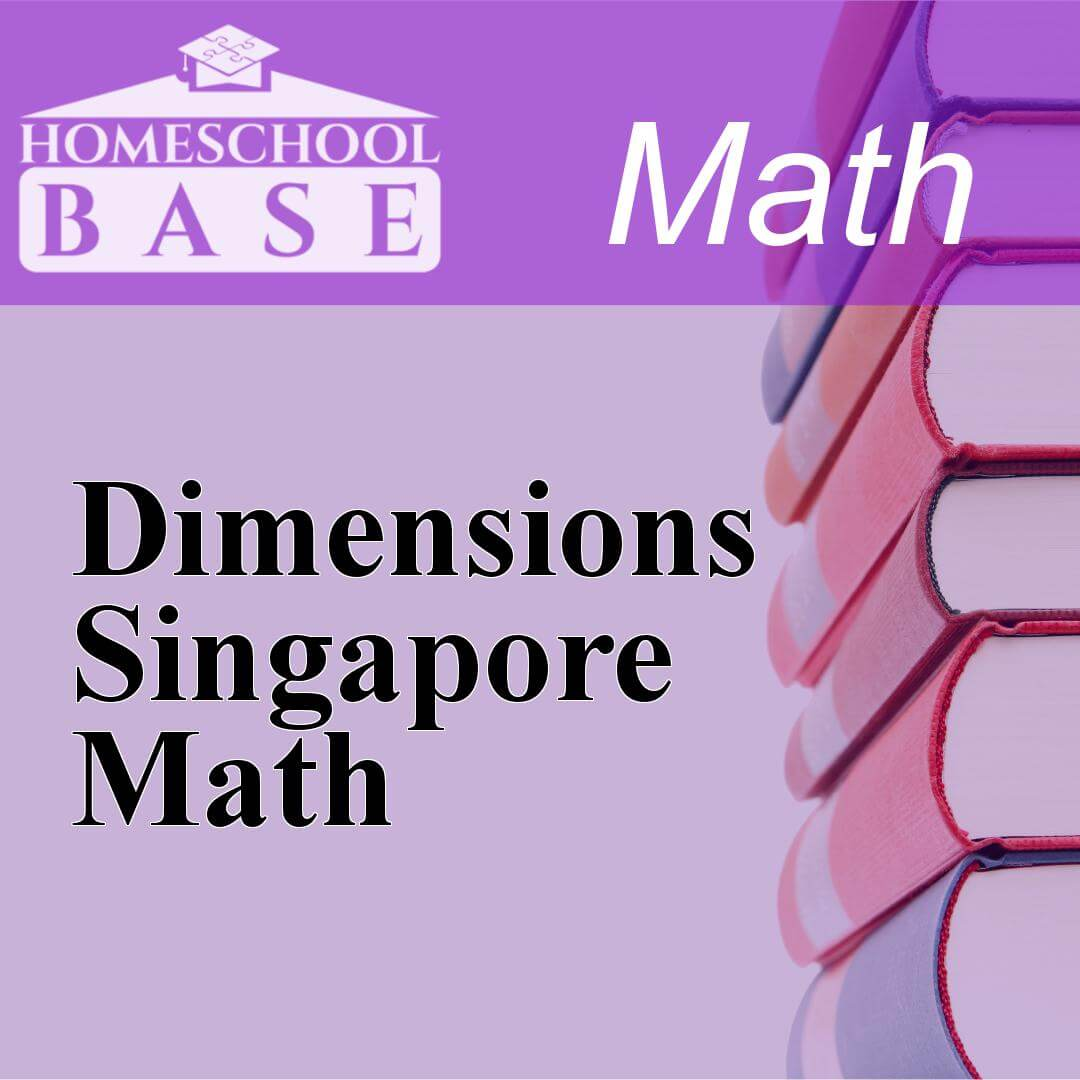## Dimensions Singapore Math

Dimensions Singapore Math is a Math curriculum for grades 7-8. This program teaches by using the sequential method. The average amount of teacher involvement on the parent(s) is: medium. This curricul...## Discovering Geometry

Discovering Geometry: An Investigative Approach is a standard geometry textbook that is popular in a few homeschooling circles. The book is most commonly used for Common Core State Standards. Therefor...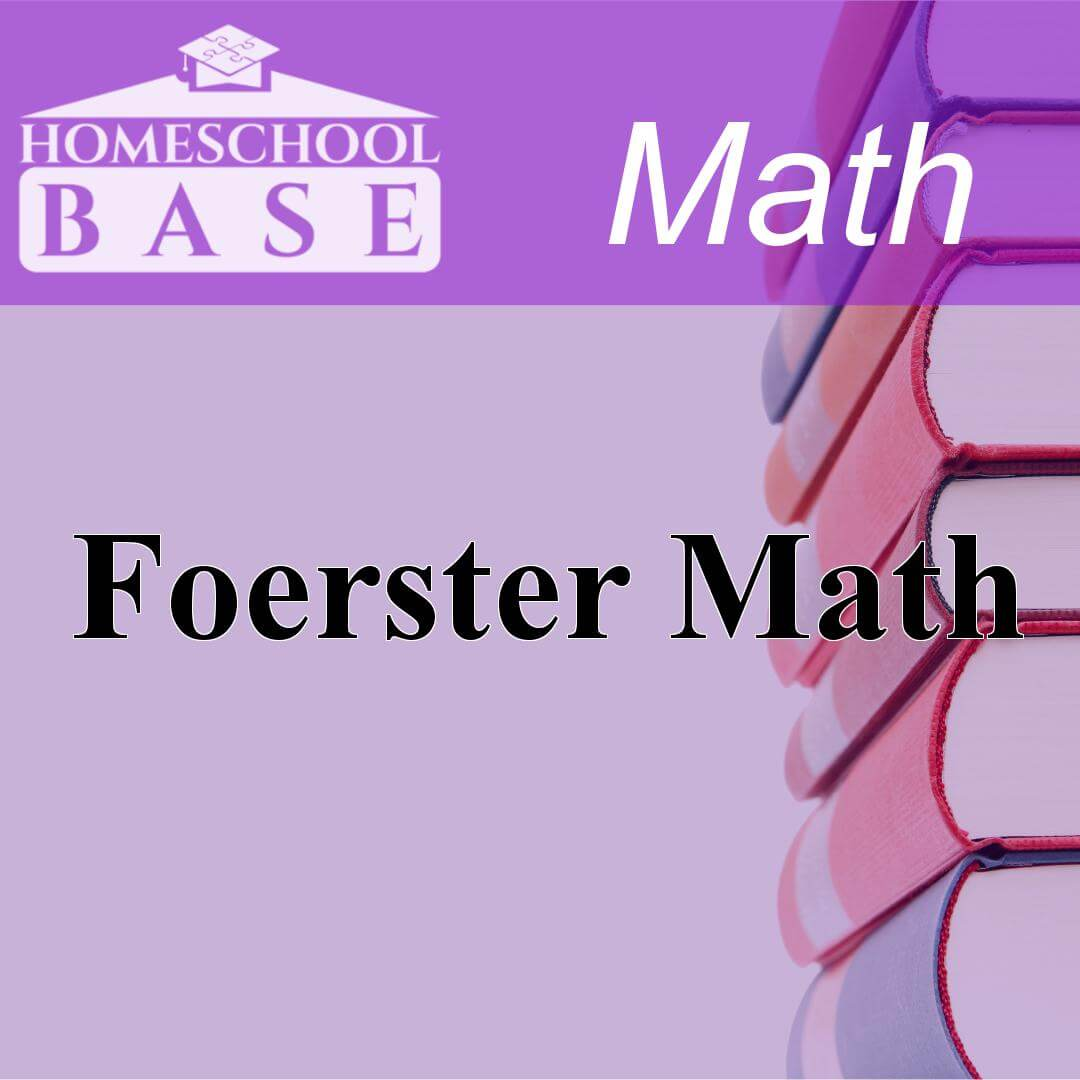## Foerster Math

Foerster Math is a Math curriculum for grades 9-12. This program teaches by using the sequential method. The average amount of teacher involvement on the parent(s) is: low. This curriculum does not co...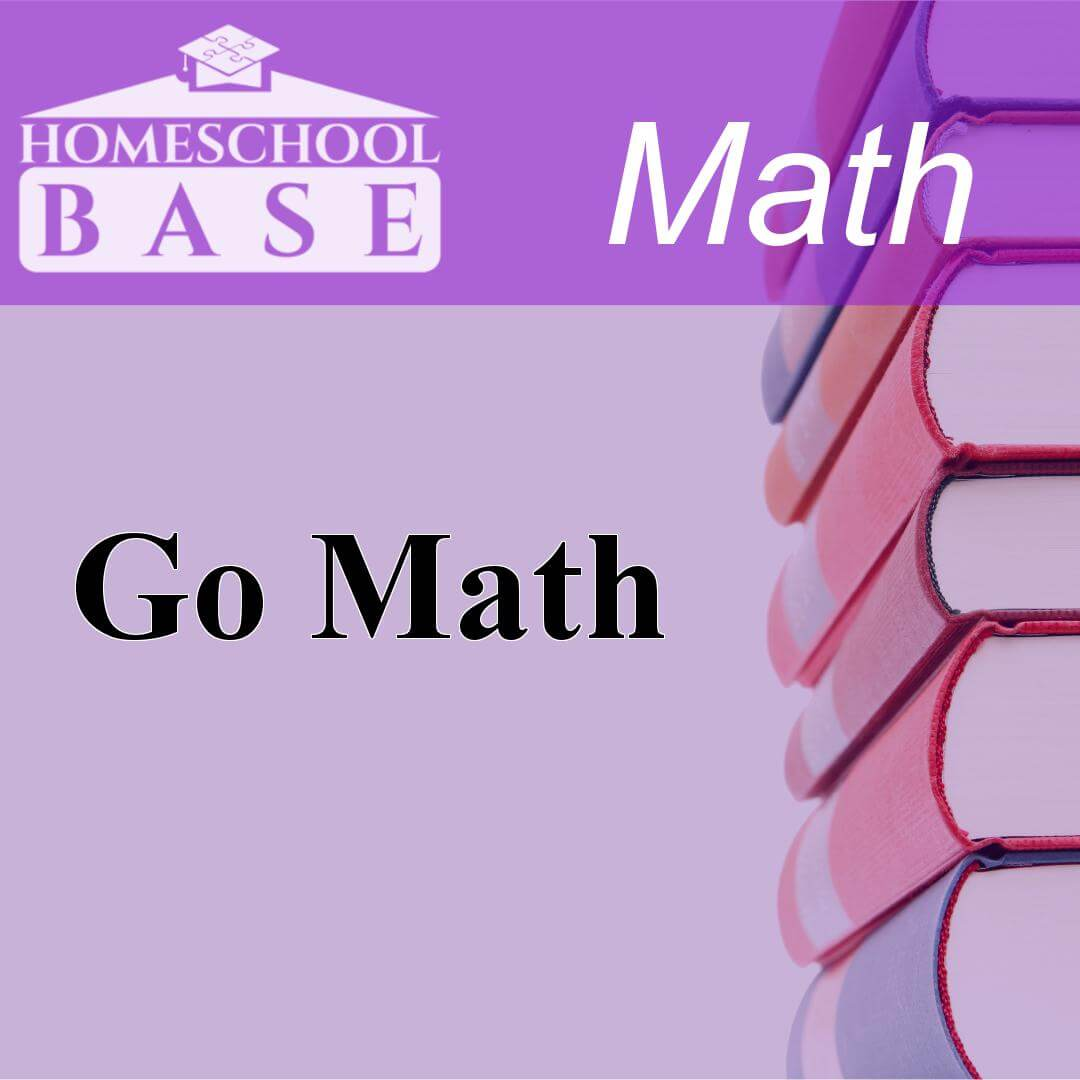## Go Math

Go Math is a Math curriculum for grades K-6. This program teaches by using the method. The average amount of teacher involvement on the parent(s) is: high. This curriculum is known to align with the ...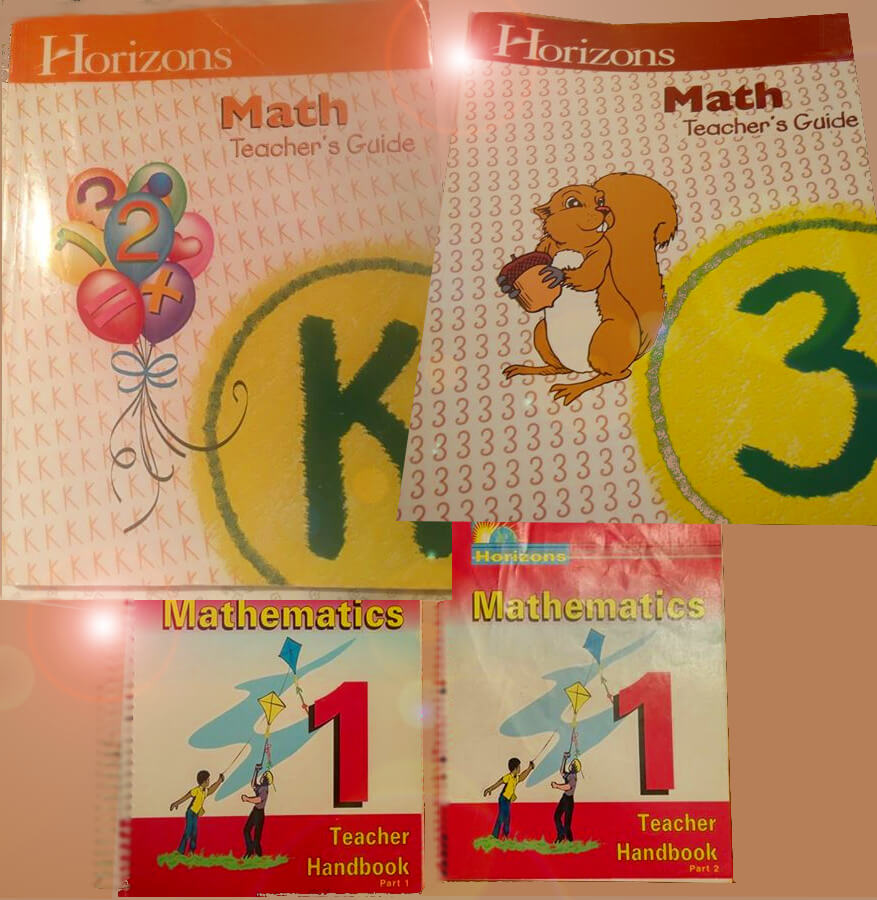## Horizons Math Curriculum

Horizons math curriculum is AOP's more "traditional approach" math program for K-8. The program makes use of manipulatives for hands-on learning. This curriculum is for homeschool parents that truly w...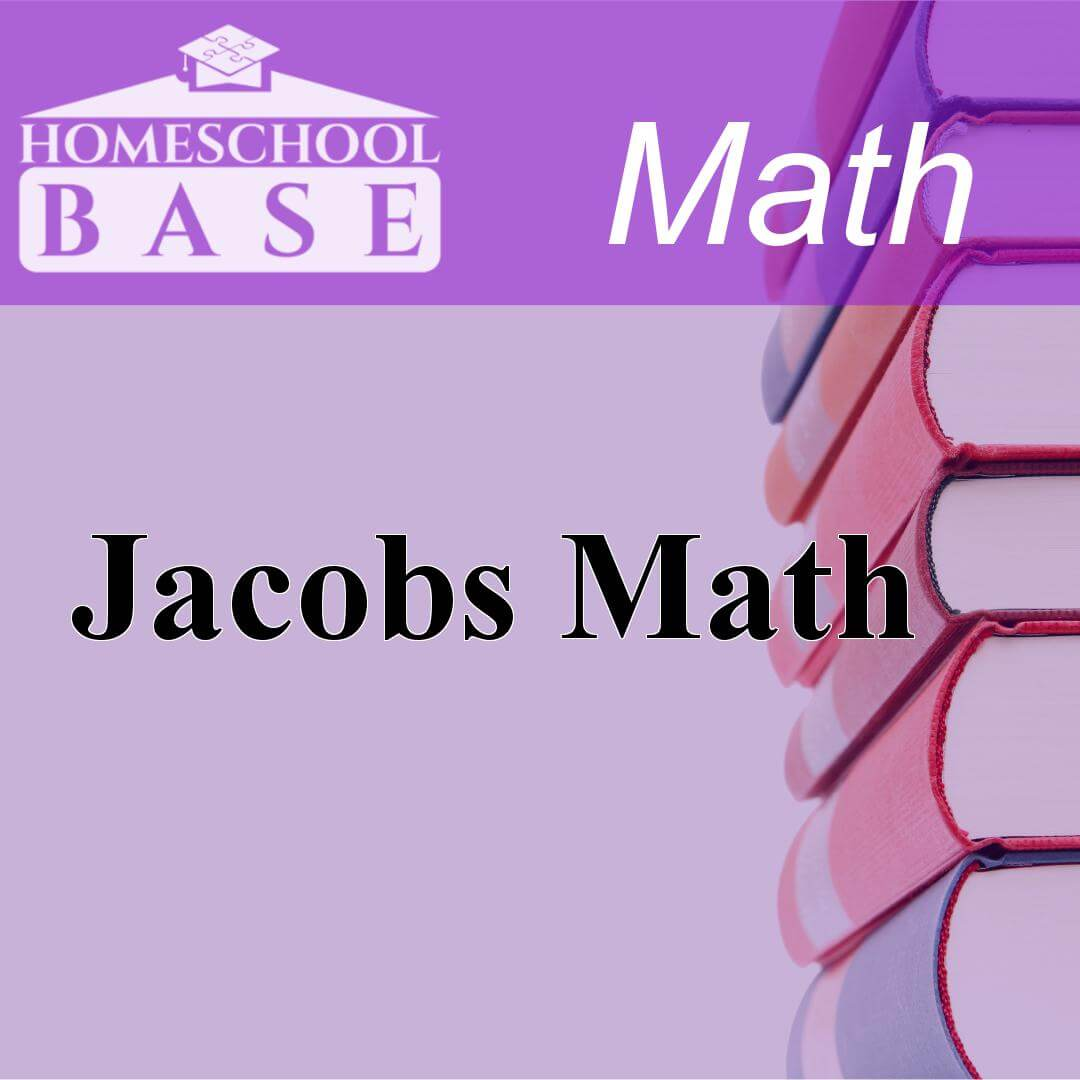## Jacobs Math

Jacobs Math is a Math curriculum for grades 9-12. This program teaches by using the sequential method. The average amount of teacher involvement on the parent(s) is: low. This curriculum does not conf...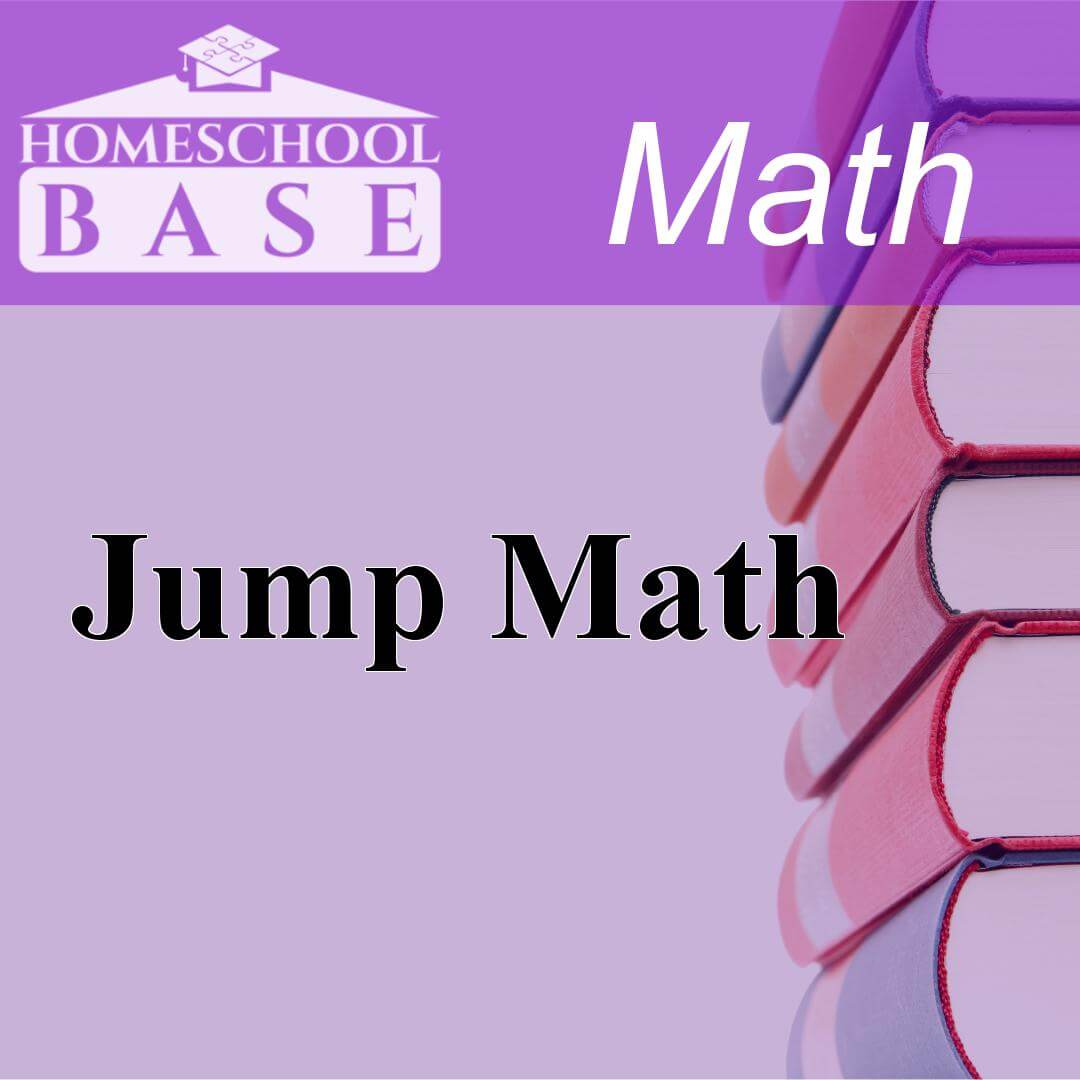## Jump Math

Jump Math is a Math curriculum for grades 1-8. This program teaches by using the sequential method. The average amount of teacher involvement on the parent(s) is: high. This curriculum is known to ali...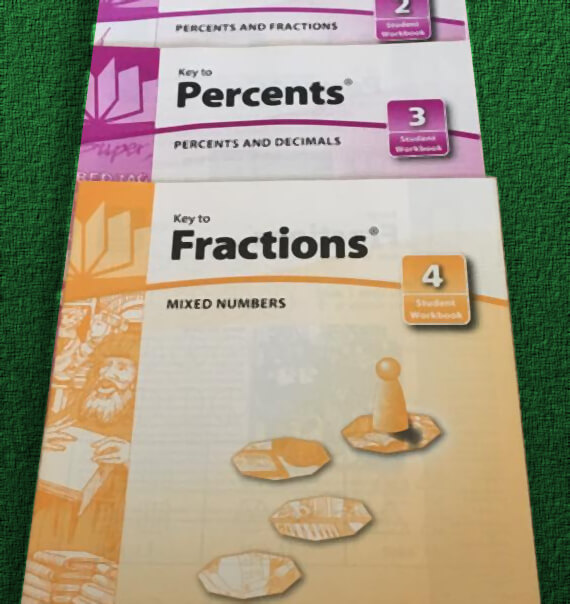## Key Curriculum Press

Key Curriculum Press publishes math curriculum like the Key to series and Miquon. Key Curriculum Press was acquired by McGraw-Hill Education in 2012. Key Curriculum Press developed inquiry-based mathe...## Key to Fractions Complete Set

Key to Fractions is a complete course on fractions for children ages 9+. Although it is not a complete math course, it is very useful for students who need extra help with fractions. The Fractions ser...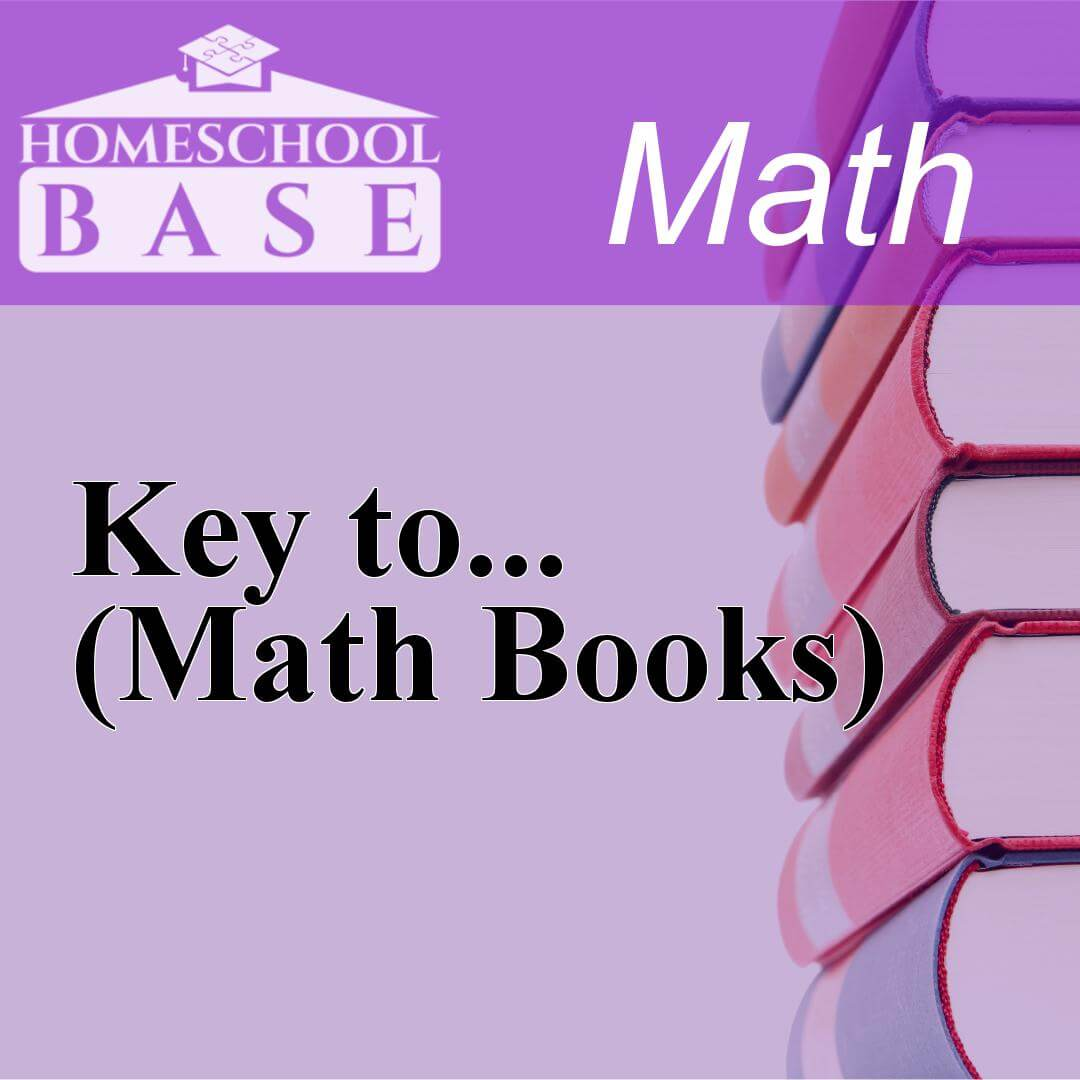## Key to... (Math Books)

Key to... (Math Books) is a Math curriculum for grades 4-12. This program teaches by using the conceptual-topical method. The average amount of teacher involvement on the parent(s) is: medium. This cu...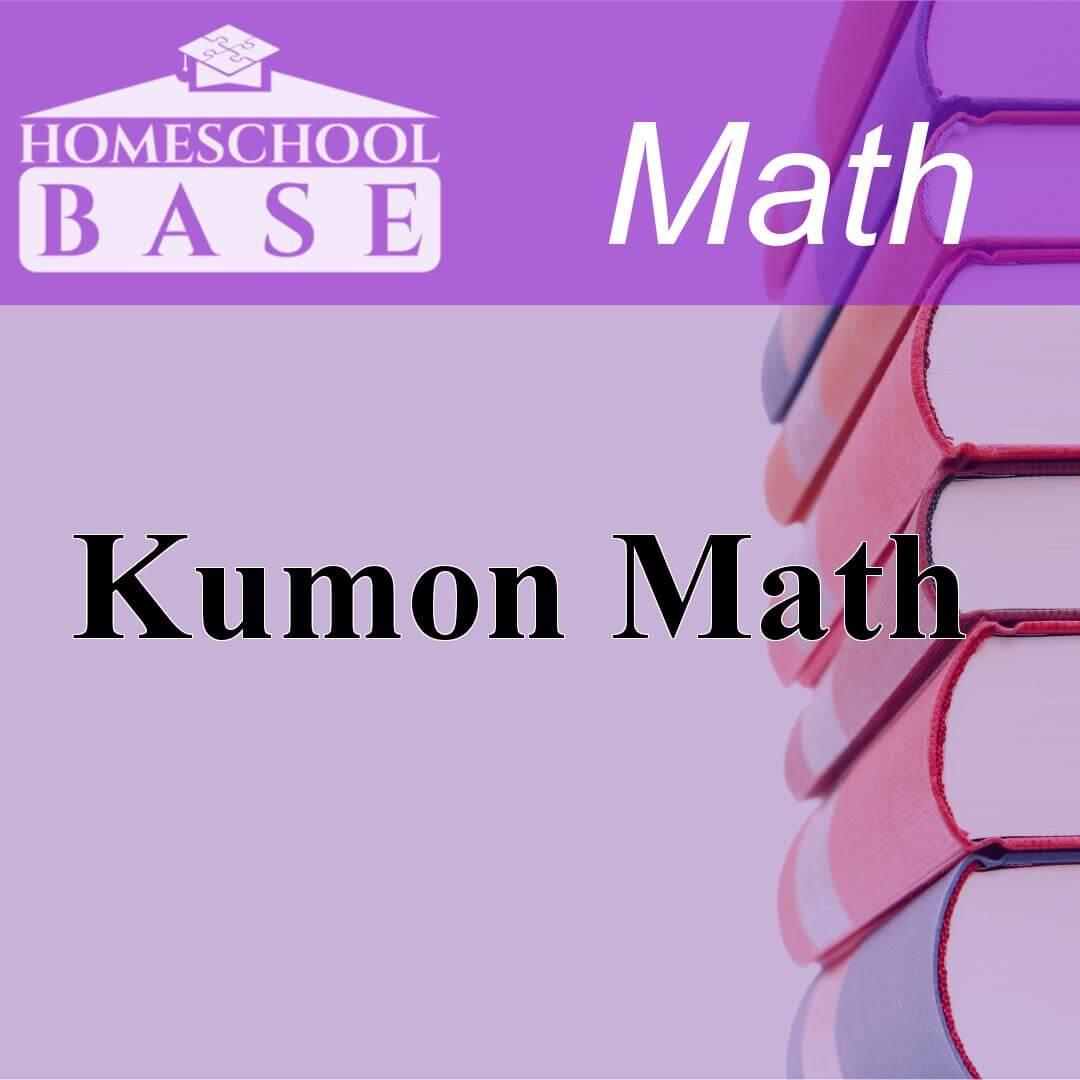## Kumon Math

Kumon Math is a Math curriculum for grades 1-6. This program teaches by using the sequential method. The average amount of teacher involvement on the parent(s) is: low. This curriculum does not confor...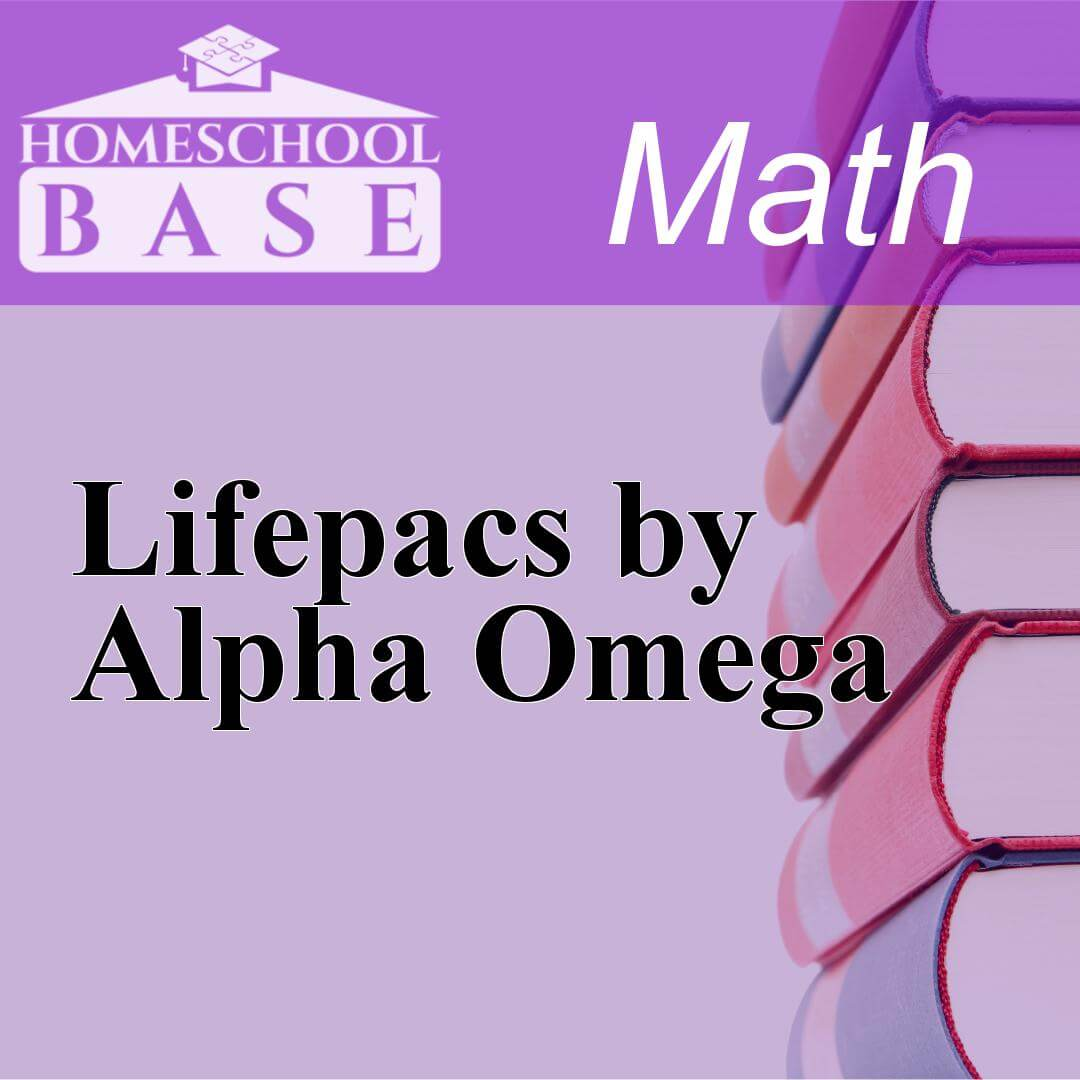## Lifepacs by Alpha Omega

Lifepacs by Alpha Omega is a Math curriculum for grades K-12. This program teaches by using the sequential method. The average amount of teacher involvement on the parent(s) is: medium. This curriculu...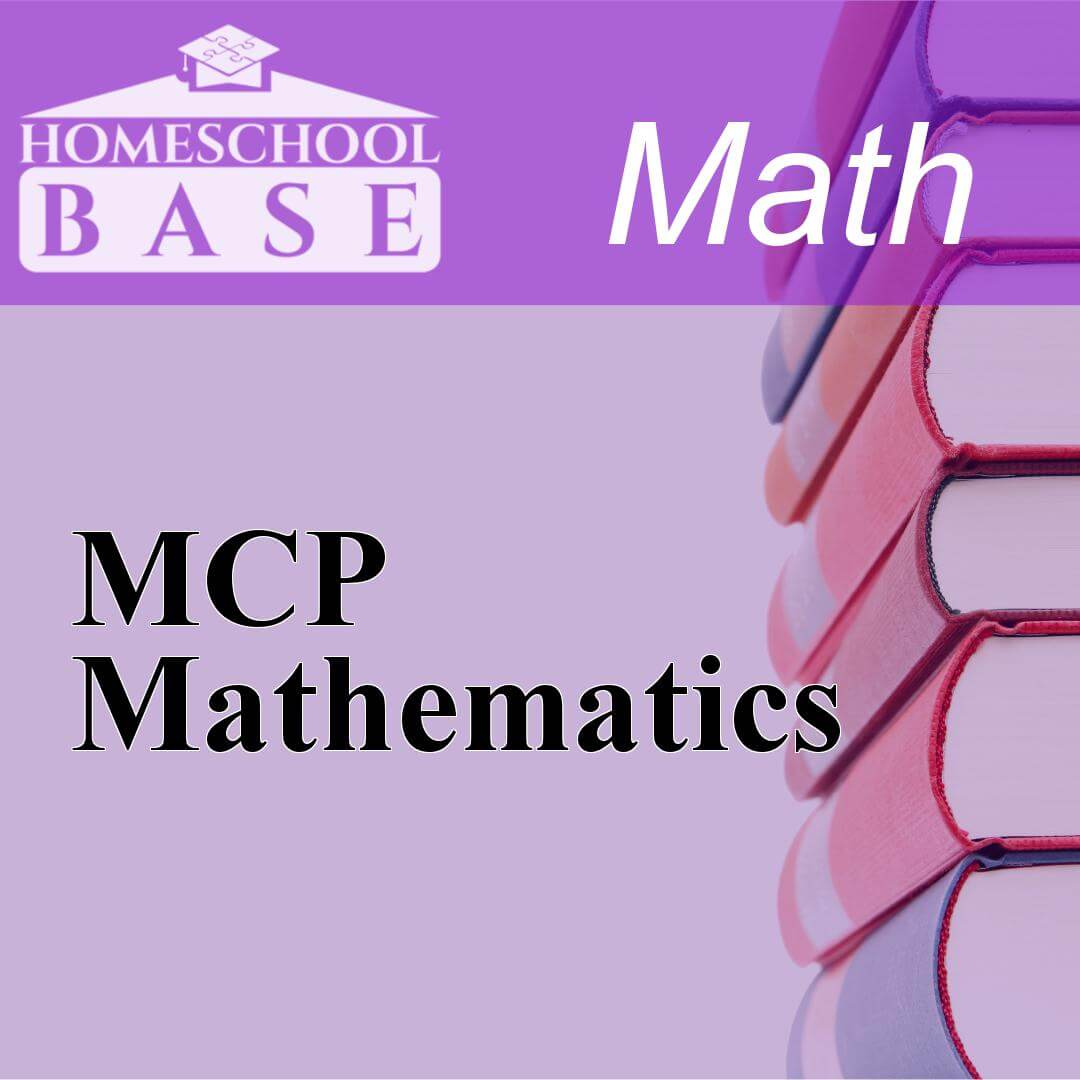## MCP Mathematics

MCP Mathematics is a Math curriculum for grades K-6. This program teaches by using the sequential method. The average amount of teacher involvement on the parent(s) is: medium. This curriculum does no...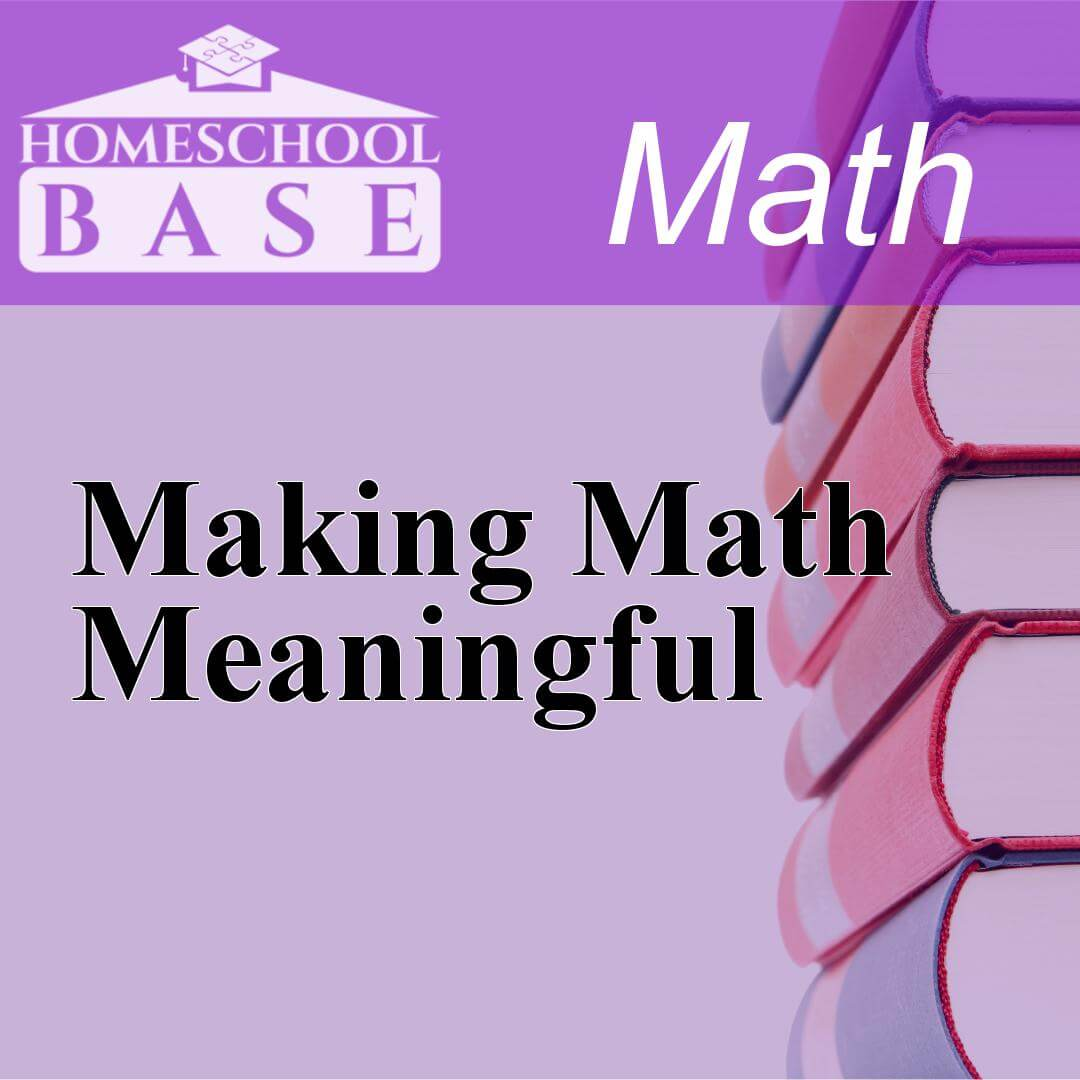## Making Math Meaningful

Making Math Meaningful is a Math curriculum for grades K-8. This program teaches by using the conceptual-topical method. The average amount of teacher involvement on the parent(s) is: high. This curri...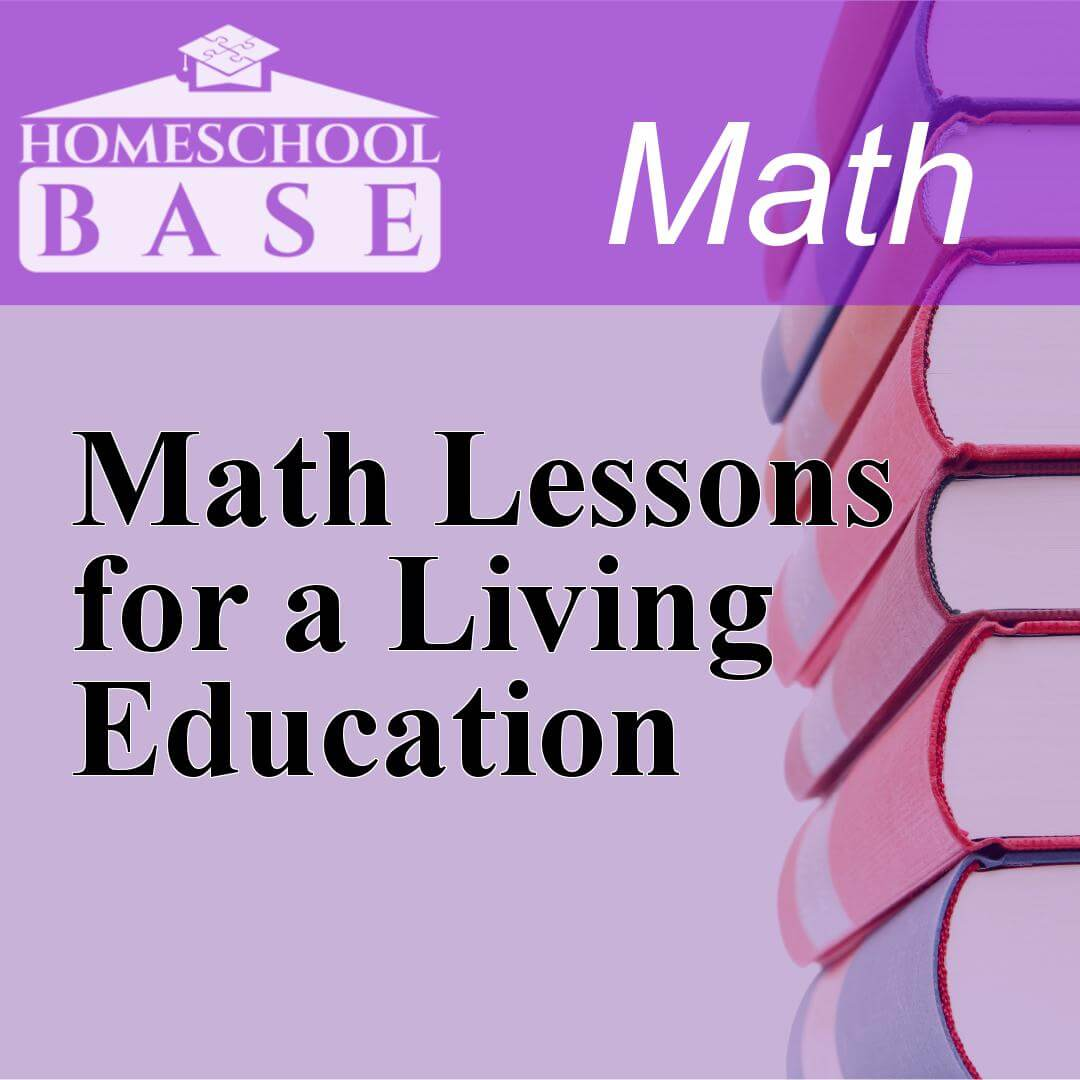## Math Lessons for a Living Education

Math Lessons for a Living Education is a Math curriculum for grades PK-5. This program teaches by using the conceptual-topical method. The average amount of teacher involvement on the parent(s) is: hi...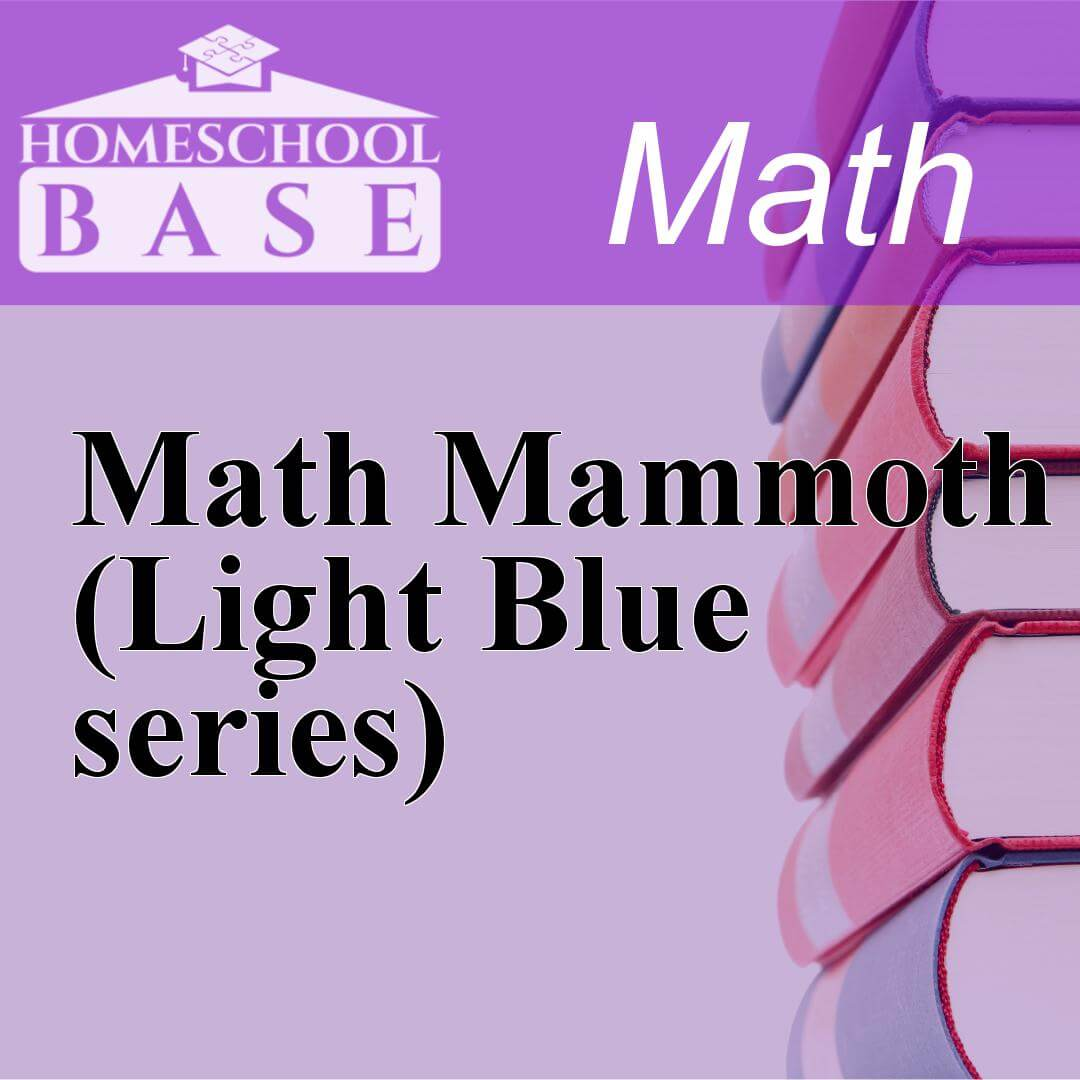## Math Mammoth (Light Blue series)

Math Mammoth (Light Blue series) is a Math curriculum for grades 1-7. This program teaches by using the sequential method. The average amount of teacher involvement on the parent(s) is: low. This cur...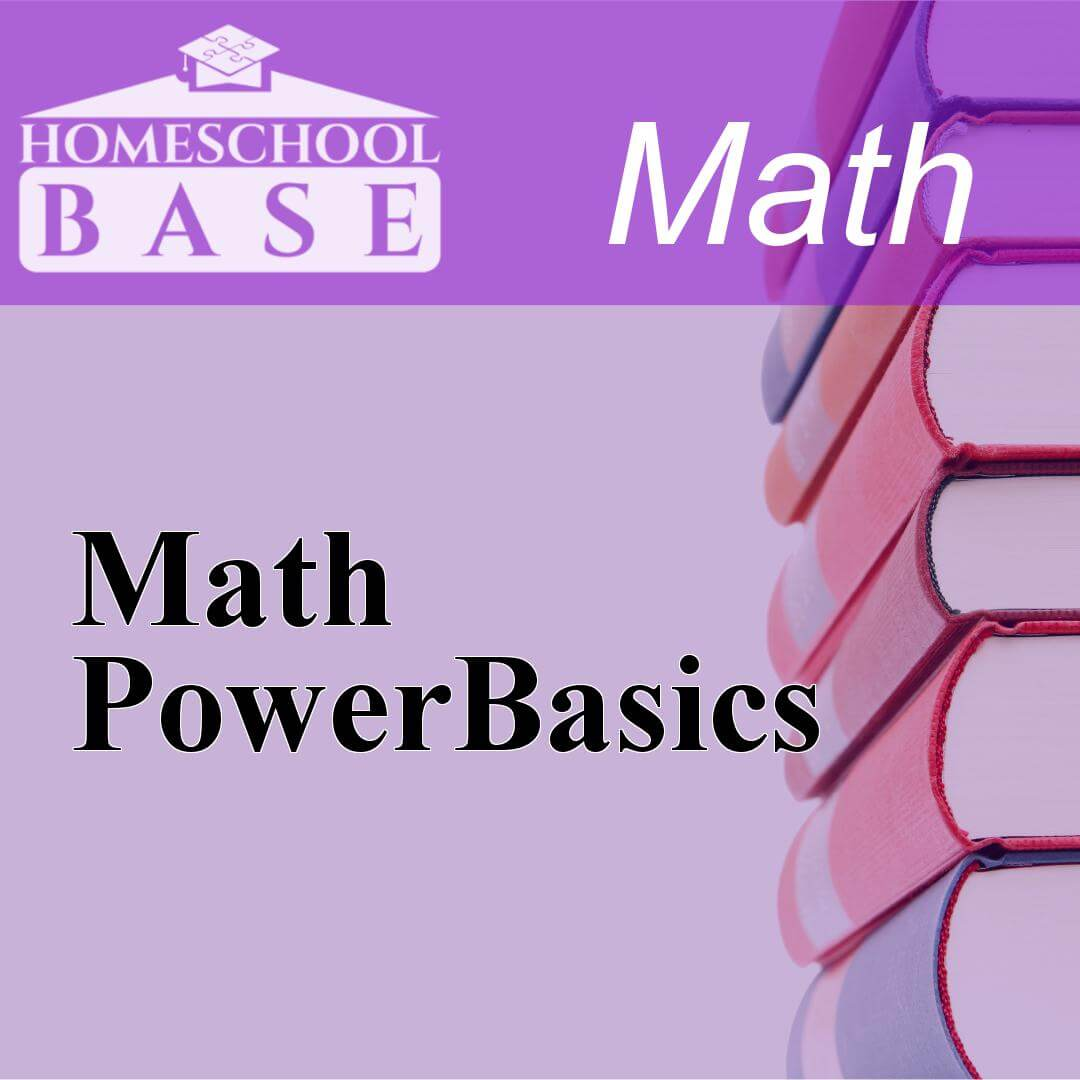## Math PowerBasics

Math PowerBasics is a Math curriculum for grades 6-12. This program teaches by using the sequential method. The average amount of teacher involvement on the parent(s) is: low. This curriculum does not...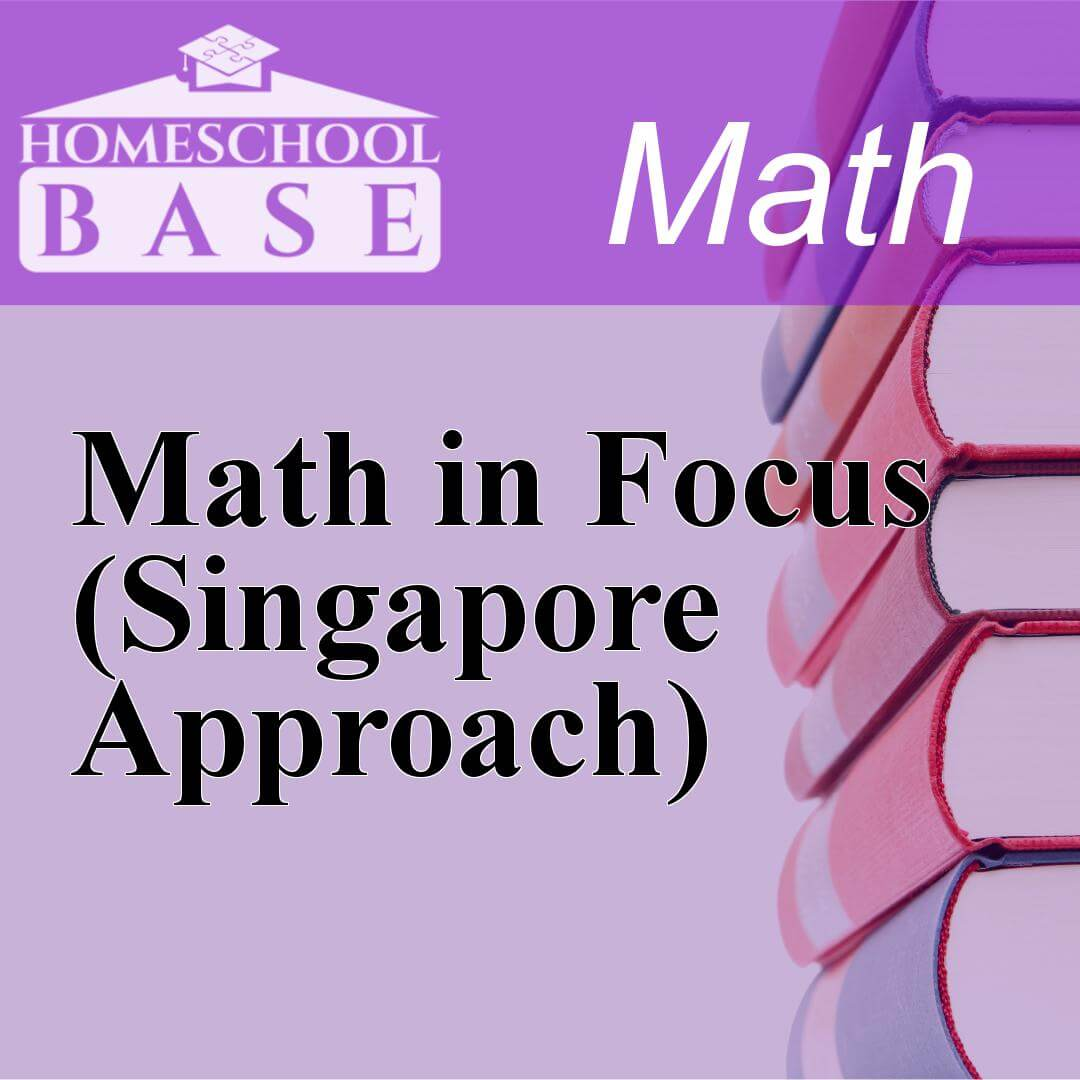## Math in Focus (Singapore Approach)

Math in Focus (Singapore Approach) is a Math curriculum for grades K-8. This program teaches by using the sequential method. The average amount of teacher involvement on the parent(s) is: medium. Thi...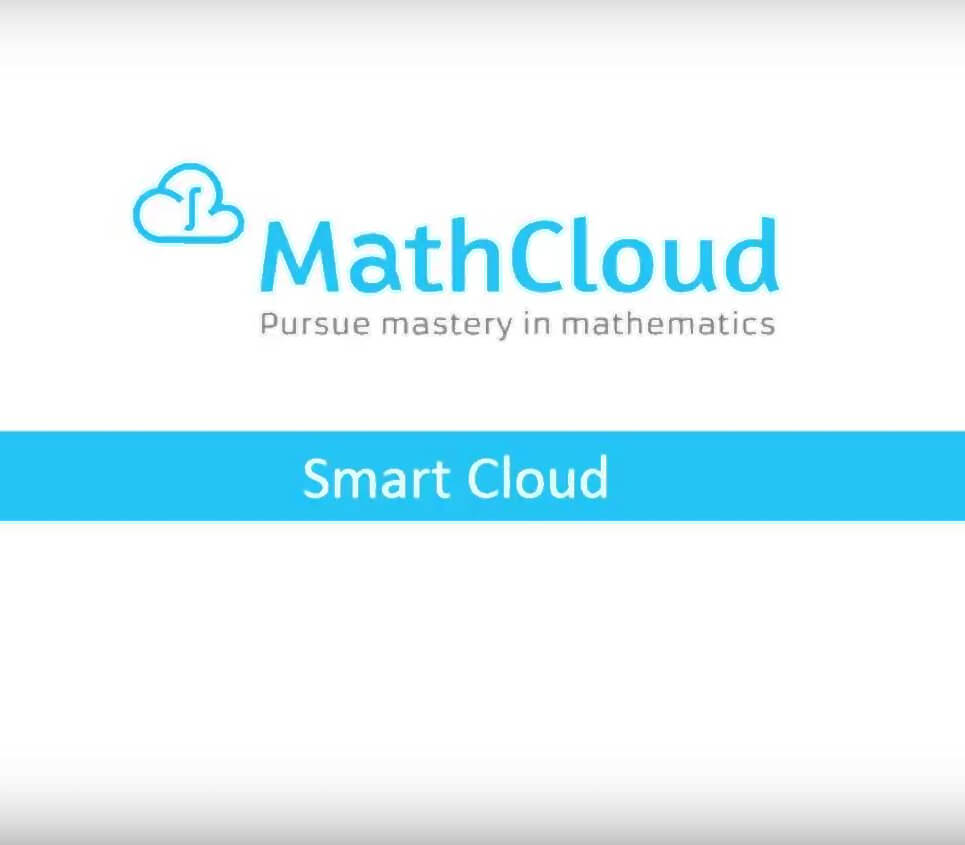## MathCloud

MathCloud offers a new approach to learning mathematics. Rather than directing students to memorize solutions and techniques, MathCloud teaches students to break complex problems down into parts and u...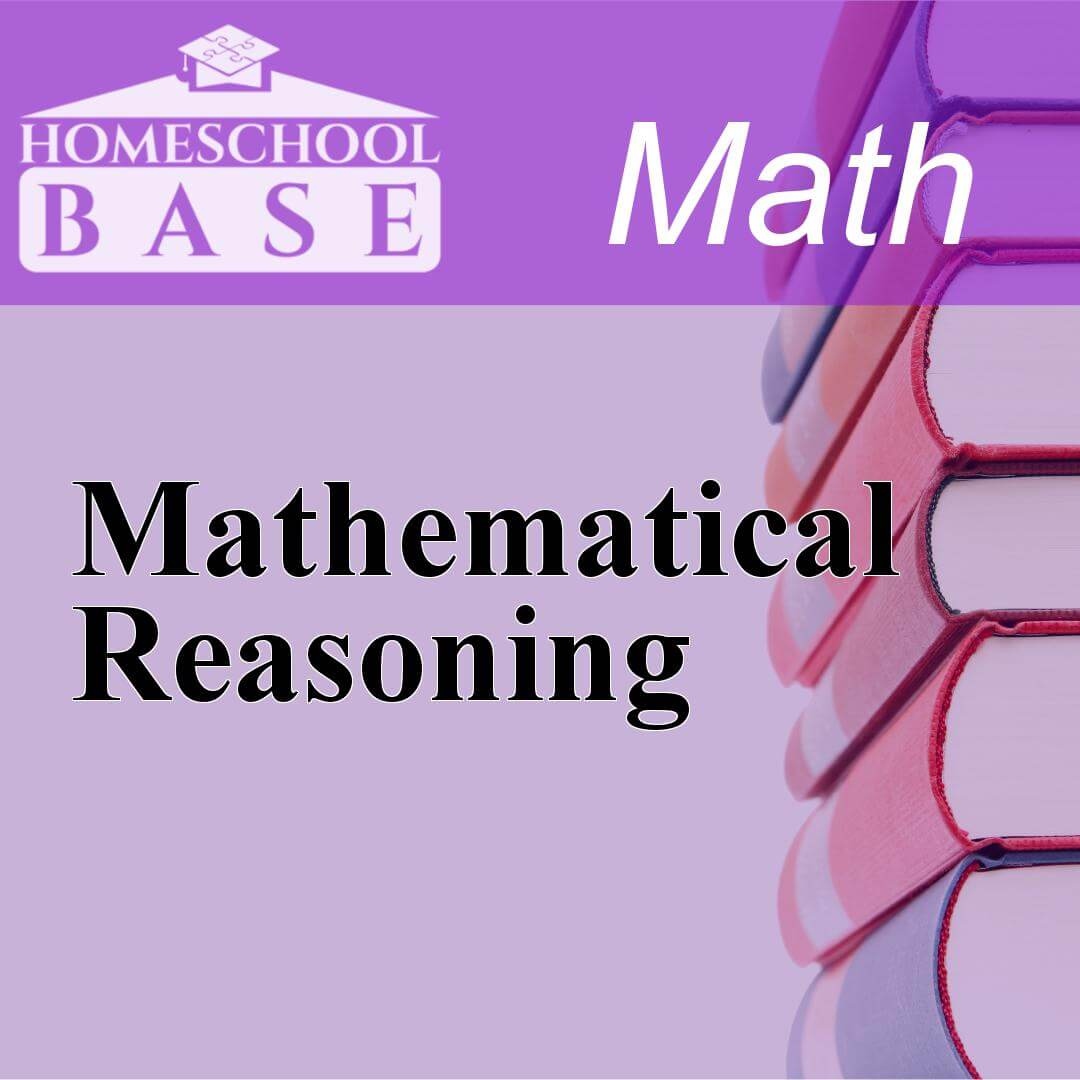## Mathematical Reasoning

Mathematical Reasoning is a Math curriculum for grades PK-9. This program teaches by using the spiral method. The average amount of teacher involvement on the parent(s) is: low. This curriculum does n...